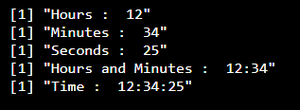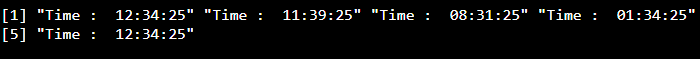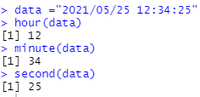GeeksforGeeks App
Open AppBrowser
Continue

# How to Extract Time from Datetime in R ?

In this article, we are going to extract time from Datetime in R programming language using lubridate() function and format() function.

Note: datetime is in the format of time and date (YYYY/MM/DD HH:MM:SS) i.e. Year:month:day Hours:Minute:Seconds

Where,

• Year:month:day comes under date
• Hours:Minute:Seconds comes under time

### Method 1: Using format() function

We are going to extract only time and for that create a variable and assign a timestamp to it. Then, extract time from the timestamp. We can do this by using as.POSIXct() function. To get a particular time hour format we can use format() function

Syntax:

format(as.POSIXct(data), format = “%H:%M”)

Parameter:

• as.POSIXct() is used to extract time from the time stamp
• format is used to get the time format. Ex : hours:Minutes and seconds
• format = “%H:%M:%S” (To get hours: minutes :seconds)
• format = “%H:%M” (To get hours: minutes )
• format = “%H” (To get hours)
• data is the time stamp

Example 1:

## R

 `# create variable with one time stamp ``data =``"2021/05/25 12:34:25"``                            ` `# get time from date using format in the ``# form of hours``print``(``paste``(``  ``"Hours : "``, ``format``(``as.POSIXct``(data), format = ``"%H"``)))`` ` `# get time from date using format in the ``# form of minutes``print``(``paste``(``  ``"Minutes : "``, ``format``(``as.POSIXct``(data), format = ``"%M"``)))`` ` `# get time from date using format in the ``# form of seconds``print``(``paste``(``  ``"Seconds : "``, ``format``(``as.POSIXct``(data), format = ``"%S"``)))`` ` `# get time from date using format in the ``# form of minutes``print``(``paste``(``  ``"Hours and Minutes : "``, ``format``(``as.POSIXct``(data), format = ``"%H:%M"``)))`` ` `# get time from date using format in the ``# form of seconds``print``(``paste``(``  ``"Time : "``, ``format``(``as.POSIXct``(data), format = ``"%H:%M:%S"``)))`

Output:Example 2:

## R

 `# create vector that stores five time stamps``data =``c``(``"2021/05/25 12:34:25"``,``"2022/05/25 11:39:25"``,``        ``"2011/05/25 08:31:25"``,``"2013/04/13 1:34:25"``,``        ``"2018/05/25 12:34:25"``)``                            ` `# get time from date using format in the``# form of seconds``print``(``paste``(``"Time : "``, ``format``(``  ``as.POSIXct``(data), format = ``"%H:%M:%S"``)))`

Output:### Method 2 : Using lubridate() function

lubridate() package is used to return the time from the datetime individually. It will return hour, minute, and second separately.

• To get hour from the datetime we will use hour command

Syntax:

hour(datetime)

Returns the time in hours

• To get minutes from the datetime we will use minute command

Syntax:

minute(datetime)

Returns the time in minutes

• To get seconds from the datetime we will use second command

Syntax:

second(datetime)

Returns the time in seconds

Example 1:

## R

 `# load the package``library``(``'lubridate'``)`` ` `# datetime variable``data =``"2021/05/25 12:34:25"`` ` `# extract hour``hour``(data)`` ` `# extract minute``minute``(data)`` ` `# extract the second``second``(data)`

Output:My Personal Notes arrow_drop_up